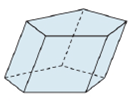Chapter 9.2, Problem 1EElementary Geometry For College St...

7th Edition
Alexander + 2 others
ISBN: 9781337614085

Solutions

Chapter
SectionElementary Geometry For College St...

7th Edition
Alexander + 2 others
ISBN: 9781337614085
Textbook Problem

In Exercises 1 to 4, name the solid that is shown. Answers are based on Sections 9.1 and 9.2.a)Bases are not regular.b)Bases are not regular.

To determine

(a)

To name:

The solid in the given figure.

Explanation

Given:

The figure given below

and bases are not regular.

Properties Used:

A prism is a polyhedron, which means all faces are flat and the side faces are parallelograms and each one is called a base. It has the same cross section all along its length.

A right prism is a prism in which the lateral edges are perpendicular to the base edges at their point of intersection.

An oblique prism is a prism in which the parallel lateral edges are oblique to the base edges at their point of intersection...

To determine

(b)

To name:

The solid in the given figure.

Still sussing out bartleby?

Check out a sample textbook solution.

See a sample solution

The Solution to Your Study Problems

Bartleby provides explanations to thousands of textbook problems written by our experts, many with advanced degrees!

Get Started

In Exercises 39-54, simplify the expression. (Assume that x, y, r, s, and t are positive.) 40. (49x2)1/2

Applied Calculus for the Managerial, Life, and Social Sciences: A Brief Approach

Evaluate each expression: 4+232

Elementary Technical Mathematics

The slope of the tangent line to r = cos θ at is:

Study Guide for Stewart's Multivariable Calculus, 8th

Evaluate 11(x2x3)dx a) 32 b) 56 c) 12 d) 23

Study Guide for Stewart's Single Variable Calculus: Early Transcendentals, 8th